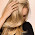KVA Rating of an Alternator

The alternators are designed to supply a specific voltage to the various loads. This voltage is called its rated terminal voltage denoted as VL. The power drawn by the load depends on its power factor. Hence instead of specifying rating of an alternator in watts, it is specified in terms of the maximum apparent power which it can supply to the load. In three phase circuits, the apparent power is √3VL IL, measured in VA (volt amperes). This is generally expressed in kilo volt amperes and is called kVA rating of an alternator where IL is the rated full load current which alternator can supply. So for a given rated voltage and kVA rating of an alternator, its full load rated current can be decided.
Consider 60 kVA, 11 kV three phase alternator.
In this case          kVA  rating  = 60

........ 10-3 to express the product in kilo volt amperes
...    60 = √3 x 11 x 103 x IL x 10-3
...    IL = 3.15 A
This is the rated full load current of an alternator. But load current is same as the armature current. So from kVA rating, it is possible to determine full load armature current of an alternator which is important in predicating the full load regulation of an alternator for various power factor conditions. Similarly if load condition is different than the full load, the corresponding armature current can be determined from its full load value.
Note : Ia at half load = 1/2 x Ia at full load. It reduces in the same proportion in which load condition reduces.
Hence regulation at any p.f. and at any load condition can be determined.

1 comment:

1.i am continually searching for some free stuffs over the web.
https://allpowersolution.com/used-mwm-generators/